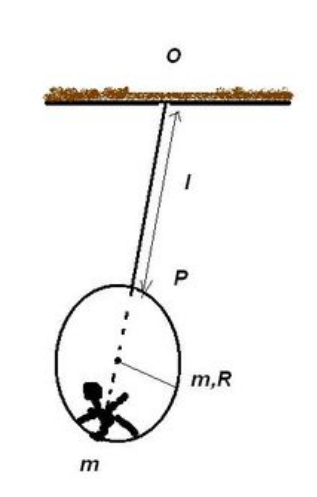The Time-keeperA man sits on the inner side of a hollow sphere and measures the seconds as they pass. Find the time (in $\si{\second}$) he measures during one oscillation of the pendulum.

Details and Assumptions

• Length of string is $l=1 \text{ m}$
• Radius of sphere is $R=1 \text{ m}$
• String is attached at point $P$ and man sits diametrically opposite to this point.
• Masses of both man and sphere are same.
• The man doesn't slip on the sphere ie., he sits at the same point on the sphere.

• Assume the figure at the bottom of the sphere to be the man.

• The oscillations take place in uniform gravitational field where $g = 9.81$ SI units.

×